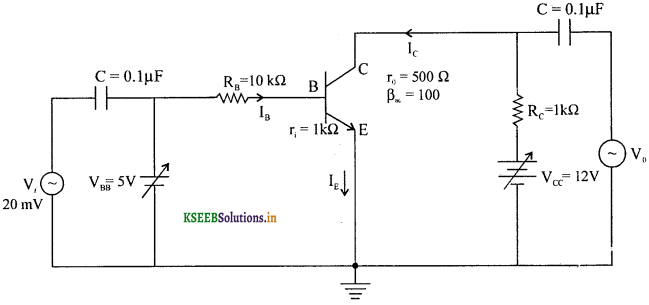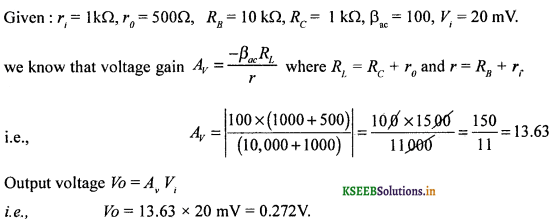# 2nd PUC Physics Question Bank Chapter 14 Semiconductor Electronics

Students can Download 2nd PUC Physics Chapter 14 Semiconductor Electronics Questions and Answers, Notes Pdf, 2nd PUC Physics Question Bank with Answers helps you to revise the complete Karnataka State Board Syllabus and to clear all their doubts, score well in final exams.

## Karnataka 2nd PUC Physics Question Bank Chapter 14 Semiconductor Electronics

### 2nd PUC Physics Semiconductor Electronics One Marks Questions and Answers

Question 1.
Give an example for a basic block of an electronic circuit.
Semiconductor transistor.

Question 2.
What is meant by basic building block of an electronic circuit?
Devices in which a controlled flow of electrons can be obtained are called basic building blocks of an electronic circuit.Question 3.
What are vacuum tube devices?
Vacuum tube devices are those devices in which copious electrons are obtained by a heated cathode and their flow is controlled by varying the anode voltage.

Question 4.
What is meant by a cathode used in a vacuum tube?
An electrode connected to the negative terminal of the D.C. source voltage is called a cathode.

Question 5.
What is meant by an anode used in a vacuum tube?
An electrode connected to the positive terminal of the D.C. source voltage is called an anode.

Question 6.
Why are vacuum tubes obsolete today?
Vacuum tube devices are bulky, consume high power, operate at high voltages and dissipate heat.

Question 7.
What is meant by a semiconductor device?
An electronic device which makes use of semiconductor materials as the building block is called a semiconductor device.

Question 8.
Name any one method of increasing the number of charge carriers in a semiconductor.
Semiconductors can be excited by light, heat or voltages so that a large number of charge carriers take part in the electrical conduction.

Question 9.
Give any one advantage of semiconductors over vacuum tubes?
Unlike vacuum tubes, semiconductors are small in size, operate at low voltages and are low power devices.

Question 10.
Name the principle on which a Cathode Ray Tube (CRT) works.
Cathode Ray Tubes work on the principle of vacuum tubes.

Question 11.
Where are CRT’s used?
CRTs are used in televisions, computers and video monitors.

Question 12.
Expand the term ‘LCD’.
LCD is an acronym for Liquid Crystal Display which works on the support of solid state devices and in place of CRT picture tubes.

Question 13.
How are solids classify based on their electrical conductivity?
Solids are classified into conductors, semiconductors and insulators.Question 14.
What is the range of resistivity of metallic conductors?
Metallic conductors possess a very high conductivity and a very low resistivity.
The range of resistivity p is front 10-12 Ωm to 10-8 Ωm. (The range of conductivity a is front 108 to 1012 Sm-1.)

Question 15.
What is the range of resistivity of semiconductors?
Semiconductors have resistivity in the range of 10-5 to 106 Ωm.

Question 16.
What is the range of resistivity of insulators?
Insulators have resistivity in the range of 1011 to 1019 Ωm.

Question 17.
Name any one elemental semiconductor.
Silicon. (The other IV gp. element is germanium).Question 18.
Name any one compound semiconductor.
Cadmium sulphate (an inorganic compound semiconductor).
Note : GaAs, CdSe, InP, GaAsP, GalnP are other examples of inorganic compound semiconductors.

Question 19.
Name any one organic semiconductor.
Anthracene (other examples are doped pthalocyanine.)

Question 20.
Name any one organic polymer semiconductor.
Polypyrrole (Polyaniline, polythiophene).

Question 21.
What is polymer electronics?
Electronic technology based on organic polymer compound semiconductors is called polymer electronics.

Question 22.
What is a valence band?
The energy band which includes the energy levels of the valence electrons is called the valence band.

Question 23.
What is a conduction band?
A band of energy which includes the energy levels of free electrons (conduction electrons) is called a conduction band.

Question 24.
What is a forbidden gap?
A band of energy levels in between the conduction band and valence band is called forbidden gap or band.

Question 25.
What is the value of forbidden gap energy of a silicon semiconductor?
The forbidden gap energy of a silicon semiconductor is 1 .leV:

Question 26.
What is the value of forbidden gap energy of a germanfum semiconductor?
The forbidden gap energy of a germanium semiconductor is 0.72eV,

Question 27.
What is the forbidden gap energy of metals?
Zero. This is because valence band overlaps with the conduction band.

Question 28.
What are holes in a semiconductor?
A hole represents a positive charge carrier and happens to be a vacant site into which an electron can recombine releasing energy in the form of light and heat.Question 29.
What are intrinsic semiconductors?
Intrinsic semiconductors are those conductors in which the number of electrons in the conduction band is equal to the number of holes in the valence band. Further, intrinsic semiconductors are undoped semiconductors.

Question 30.
How does an extrinsic semiconductor crystal maintain charge neutrality?
In an extrinsic semiconductor crystal, the charge of additional charge carriers is just equal and opposite to that of the ionised cores in the lattice.

Question 31.
Relate number densities of holes and electrons with the number density of ionised atoms.
ne nh = n2
where, ne – number density of electrons,
nh – number density of holes and
ni – number density of ionised atoms.

Question 32.
Mention the forbidden gap energy corresponding to diamond.
The forbidden gap energy for diamond is 5.4 eV.

Question 33.
Mention the forbidden gap energy of insulators.
The forbidden gap energy of insulators is greater than 5 eV.

Question 34.
Name the majority charge carriers in N-type semiconductors.
Electrons in the conduction band are the majority charge carriers in N-type semiconductors.

Question 35.
Name the majority charge carriers in P-type semiconductors.
Holes in the valence band are majority charge carriers in P-type semiconductors.

Question 36.
What is the net current in a p-n junction under equilibrium?
Zero.Question 37.
Mention the thickness of depletion region in a p-n junction.
The thickness of depletion region in a p-n junction is about one micron (one micron = 1 micrometre).

Question 38.
What type of charges are left near and on either sides of the junction diode?
Immobile charges. An ionised acceptor (negative charge) will be left in the p-type and ionised donor (positive charge) in the n-type during the diffusion of majority charge carriers across the p-n junction.

Question 39.
When is a diode said to be forward biased?
A diode is said to be forward biased when the p-side is connected to the positive end of a battery and the n-side is connected to the negative end of it.

Question 40.
What is the reverse bias resistance of an ideal junction diode?
Infinity.

Question 41.
What is the forward bias resistance of an ideal junction diode?
Zero.

Question 42.
Name a device which converts ac into dc.
Rectifier.

Question 43.
What is the value of cut-in or threshold voltage for a germanium diode?
The cut in voltage for germanium diode is 0.25 V. (For silicon-diode the cut in voltage is 0.65 V).

Question 44.
What is a half-wave rectifier?
A device that converts a bidirectional current into an unidirectional current is known as a half-wave rectifier.

Question 45.
What is meant by a Full Wave Rectifier?
A rectifier which responds to both the halves of the applied bidirectional current is called a Full Wave Rectifier.

Question 46.
Compare the Vpeak due to HWR and FWR.
(Vp)FWR = 2(Vp)HWR and (Vp)HWR = $$\frac{V_{0}}{\pi}$$

Question 47.
Why is a filter circuit used in rectifier circuits?
A filter circuit is used to eliminate a-c ripples in the output voltage across the load resistance.

Question 48.
Mention any one type of filter circuit that can be used to eliminate ac ripples in the output voltage.
Capacitor filter and inductive filter.Question 49.
Draw a neat labelled diagram of the output of a HWR.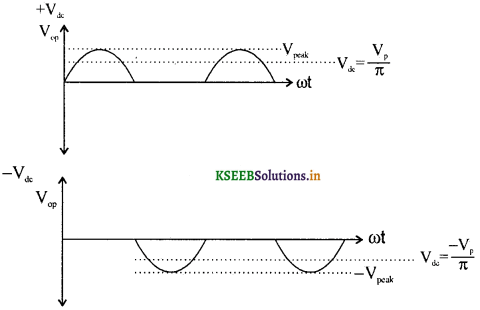The output waveforms depend on the connectivity of P-type or N-type to one end of the transformer.

Question 50.
Draw a neat labelled diagram of the output of a FWR.Question 51.
What is a Zener diode?
A zener diode is a junction diode made to operate under reverse bias in the breakdown region.

Question 52.
Name any two devices which can be used as voltage regulators.
Zener diodes and Light emitting diodes can be used as voltage regulators.

Question 53.
Mention the order of electric field in the depiction region in an unbiased junction diode.
For a thickness of one micron and for a silicon diode of voltage 0.7V, electric field will be
$$\frac{0.7}{10^{-6}}$$ = 0.7 × 106 Vm-1 ≈ 1.0 × 106Vm-1.
Hence the order of the field in the depletion region is 106Vm-1.

Question 54.
Name any one optoelectronic junction device.
Photodiodes. (Other devices are LEDs, photovoltaic cells).

Question 55.
What are photodiodes? (March 2015)
Photodiodes are junction diodes, which are operated at reverse bias mode. When light falls on it, minority charge carriers conduct electricity.Question 56.
What are photodiodes used for? (March 2015)
Photodiodes are used as photodetectors as in the case of IR detector in a DVD system and TV system. (Photo diodes are also used in burglar alarms, IR detector, Ere detector etc.)

Question 57.
What are LEDs?
When conduction electrons fall into the holes near the junction, energy is liberated. This energy’ is radiated as visible light or infra red radiations depending upon the type/of semiconductors used.
These types of diodes are called LEDs.

Question 58.
What is a transistor?
A transistor is a three-terminal two junction device comprising of emitter, base and collector regions.

Question 59.
What is the type of bias b/w CB and EB for normal operation?
In a normal operation, CB is reverse biased and EB is forward biased.

Question 60.
Which one of the three regions of a transistor is heavily doped?
Emitter region.

Question 61.
Why is the collector region doped, at intermediate level compared to the emitter and the base?
The collector region collects a major portion of the majority carriers from the emitter. Hence ” the collector is moderately doped and has a larger size than the emitter.

Question 62.
Expand the term transistor.
Transistor refers to Transfer Resistor. (Unlike in conductors, the flow of electrons will be from a low resistive path to a high resistive path and conventional current called hole current will be from a high resistive path to a low resistive path.

Question 63.
Draw input characteristic curve for a CE transistor circuit.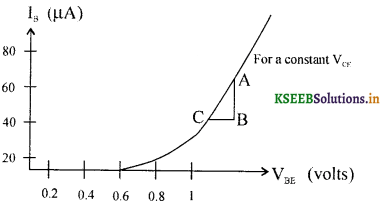Question 64.
What does the slope in the linear region of input characteristic curve determine?
Input resistance, rin = $$\left(\frac{\Delta \mathrm{V}_{\mathrm{BE}}}{\Delta \mathrm{I}_{\mathrm{B}}}\right)_{\mathrm{v}_{\mathrm{ck}}}$$Question 65.
What does the ratio $$\left(\frac{\Delta \mathbf{V}_{\mathbf{C E}}}{\Delta \mathbf{I}_{\mathbf{C}}}\right)$$ for a constant IB determine?
Output resistance is r0 = $$\left(\frac{\Delta \mathrm{V}_{\mathrm{CE}}}{\Delta \mathrm{I}_{\mathrm{C}}}\right)_{\mathrm{I}_{\mathrm{B}}}$$

Question 66.
Define ac signal current amplification factor (β).
Current amplification factor p may be defined as the ratio of the small change in the collector current to the small change in the base current at a constant voltage b/w collector and emitter.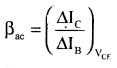Question 67.
Define βdc of a transistor.
The ratio of collector current to base current is called βdc of a transistor.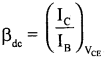Question 68.
What will be the value of Pdc for most of the transistors?
βdc > 20 for most of the transistors.

Question 69.
What is a switch?
Switch is a device which can allow or disallow the flow of charge in a circuit.

Question 70.
In which regions of transfer characteristic does a transistor work as a switch?
The transistor is made to remain either in the cut off region or in the saturation region but no in the active region to act as a switch.Question 71.
What will be the voltage between the collector and the emitter (VCE) of a transitor, used as a switch, during the cut off state?
The voltage between the collector and the emitter will be equal to Vcc during the cut-off. Note: During the cut-off state, collector current becomes zero and no voltage drop across the load resistor. Hence the two terminals of the resistor will be driven to same potential as Vcc.

Question 72.
What will be the voltage between VCE during the saturation region?
Almost zero.
Note: During saturation, collector of the transistor will be at the same zero potential as that of the emitter.

Question 73.
What is an amplifier?
An amplifier is a device which increases the amplitude of the input signal.

Question 74.
What is amplification?
A small change in base current produces a large variation in the collector current. This property of an amplifier is known as amplification.

Question 75.
What does the negative voltage gain of a CE amplifier indicate?
The negative voltage gain indicates that the output voltage is opposite in phase with the input voltage.

Question 76.
Give the expression for power gain of a CE amplifier.
Power gain = (voltage gain) (current gain) = βacAv = -βac2 (RC/RB).

Question 77.
What type of feedback is found ip the amplifier circuit?
Negative feedback.

Question 78.
What do you call an amplifier with a positive feedback?
Oscillator.

Question 79.
What is meant by a positive feedback?
A portion of the output power is returned back to the input in phase with the input (starting) power. This type of feedback is called as positive feedback.

Question 80.
Write the expression for the frequency of an LC oscillator?
Frequency f = $$\frac{1}{2 \pi \sqrt{L C}}$$ Hz where, L = self-inductance of the coil and C = electrical capacitance.

Question 81.
What is a binary signal?
A binary signal has two levels of voltage called zero state (0 V) and one state (+5V). The combination of the voltage variations between these two voltage levels is called as a binary signal.

Question 82.
What are logic gates?
Logic gates are electronic circuits which make logical decisions.

Question 83.
What is a logic OR gate?
A logic OR gate is that gate whose output will remain low if and only if all the inputs are low.Question 84.
Write the truth table for logic OR gate. (March 2014)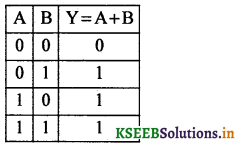Question 85.
What is a logic AND gate?
Logic AND gate is that logic gate whose output will be high if and only if all the inputs are high.

Question 86.
Write the truth table of AND gate.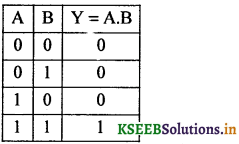Question 87.
What is an INVERT or NOT gate?
A logic gate whose output is negation or complement of the input is known as NOT or INVERT gate.

Question 88.
What is a NAND gate?
ANAND gate is a negated AND gate in which the output will remain low if and only if both the inputs are high.
Y = $$Y=\overline{A B}=\bar{A}+\bar{B}$$

Question 89.
Write the truth table of NAND gate.Question 90.
What is a NOR gate?
A negated OR gate in which the output will remain high if and only if both the inputs are low is called a NOR gate.

Question 91.
Write the truth table of NOR gate.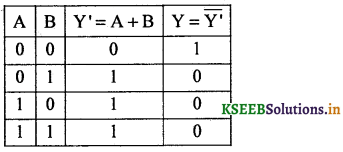Question 92.
Mention any one type of integrated circuit.
Linear or analogue ICs, in which the output is proportional to the input.
Note: The other type of IC is digital IC which is non-linear.Question 93.
Give an example for a linear IC.
Operational amplifier is an example for a linear IC.

Question 94.
What is an integrated circuit?
An electric circuit which fabricated on a single block or chip comprising of active and positive components is called an integrated circuit.

Question 95.
Mention any one application of LEDs.
LEDs are used as display units, power indicators.

Question 96.
What is a solar cell?
Solar cell is an opto electrical device which converts light energy into electrical energy.

Question 97.
Mention two necessary conditions for doping.

1. The size of the dopant atom should be almost the same as that of the semiconductor atom.
2. The dopant atoms should not distort the crystal lattice.### 2nd PUC Physics Semiconductor Electronics Two Marks Questions and Answers

Question 1.
Represent a NOT gate using a NAND gate or a NOR gate.
1. NOT gate using a NAND gate:2. Not gate using a NOR gate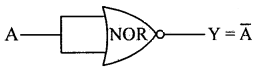Question 2.
Mention any two ways of increasing the conductivity of an intrinsic semiconductor.
1. In the first method, the conductivity can be increased in Silicon or Germaniun, by increasing the hole population, by doping or adding a trivalent impurity atom such as Boron (B), Aluminium (Al), Gallium (Ga) or Indium (In).

2. In the second method, the conductivity can be increased in Silicon or Germaniun, by increasing the electron population by doping with a pentavalent impurity atom such as phosphorous (P), Arsenic (As) or Antimony(Sb).

Question 3.
What are p-type and n-type semiconductors?
Ge or Si doped with any one of the trivalent atoms like Ba, Ga, Al and In, is a p-type semiconductor.
Ge or Si doped with any one of the pentavalent atoms like P, As, Sb, Bi is a n-type semiconductor.

Question 4.
Mention the two methods of biasing a diode.
The two methods of biasing a diode are:

1. Forward Bias: P side is connected to the positive terminal and N side to the negative terminal of the voltage source.
2. Reverse Bias: P side is connected to the negative terminal and N side to the positive terminal of the voltage source.

Question 5.
Distinguish between p-type and n-type semiconductors. (July 2014)Question 6.
Distinguish between n-p-n and p-n-p transistors.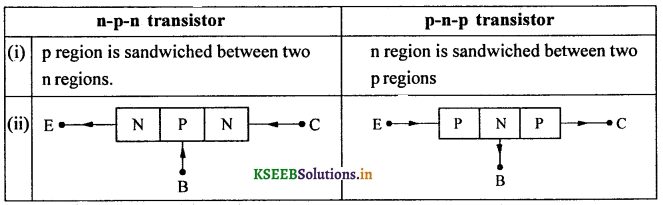Question 7.
What is a photo diode? In which mode of biasing does it work?
A photo diode is an opto – semiconductor device which works when light falls on it. Photo diode works on reverse bias mode.

Question 8.
Relate IE, IB, and Ic for a transistor satisfying DC condition.
IE = IB + IC
where, IB – Base current, Ic – Collector current, IE – Emitter current
and IE = Ih + Ic
where, Ih = hole current
and Ie – electron current.

Question 9.
Define current gain at a constant VCE and VBE.Question 10.
What is meant by input and output resistance of a transistor amplifier n CE mode?
1. The dynamic or ac input resistance is defined as the ratio of change in base- emitter voltage to the change in base current at a constant collector-emitter voltage.2. The dynamic or ac output resistance is defined as the ratio of change in collector – emitter voltage to the change in collector current at a constant base current.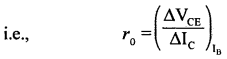Question 11.
Mention a few semiconductors employed in the fabrication of solar cells.
Silicon (Eg = 1. leV), GaAs (Eg = 1,43eV), CdTe (Eg = 1.45 eV) and CuInSe2 (Eg = 1.04eV).

Question 12.
Explain the working of a typical p-n junction solar cell with a neat labelling.
Working of a solar cell: When light of suitable wavelength is incident on an open-circuited solar cell, an emf is produced across its terminals. Either p or n is made very thin so that a large light energy is not absorbed by the thin region. The junction area is kept large so that a large amount of solar radiation falls on it. The electrons reaching the n-side are collected by the front contact and holes reaching the p-side are collected by the back contact. Thus, p-side becomes positive and inside becomes negetive giving rise to photo voltage.
Isc – Short circuit current VQC- Open circuit voltageQuestion 13.
Draw I-V characteristics of a solar cell.Question 14.
Draw a labelled diagram for a N-P-N transistor showing the necessary DC-bias conditions.Question 15.
Draw DC bias condition for a PNP transistor.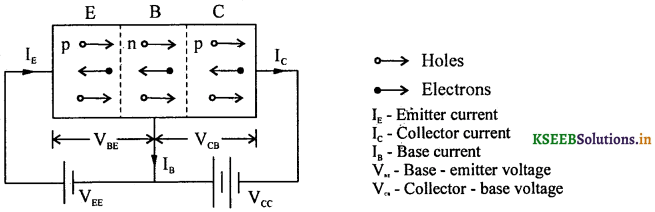Question 16.
Show the circuit arrangement for studying the input and output characteristics of a n-p-n transistor in CE configuration.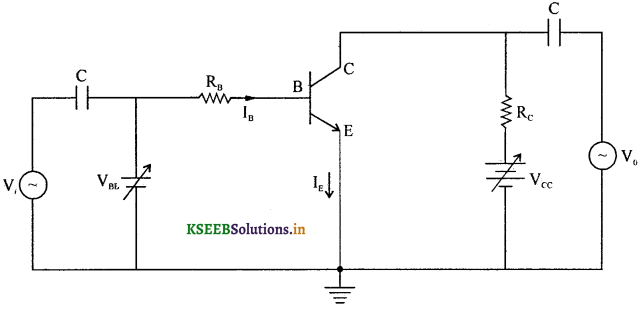Question 17.
Draw a neat labelled diagram of a transistor amplifier in a CE mode.Question 18.
Explain briefly Barkhausen’s criteria.
If voltage feedback Vfb is derived from the output voltage V0 such that the fraction of feedback is β’ then Vfb = β’V0.
Let ‘A’ be the amplification such that
A(β’V0) = V0.
Hence, β’A = 1. This is the condition for stable oscillation and is called Barkhausen’s criteria.Question 19.
Distinguish between positive feedback and negative feedback.
If the feedback voltage is in the same phase to that of the input voltage, then the feedback is said to be positive

If the feedback voltage is in the opposite phase to that of the input voltage, then the feedback is said to be negative.

An amplifier with a positive feedback is a stable oscillator in which the gain is more than one.
A transistor circuit with a negative feedback is called as an amplifier and whose gain is less than one and can never work as an oscillator.

Question 20.
What is Boolean algebra?
George Boole developed an algebra to solve the logical problems, which is known as Boolean algebra. This Logic allows only two values or states for a variable either ‘true’ or ‘false’.

Question 21.
Give the logic symbol, Boolean expression and truth table of an AND gate.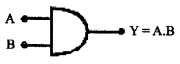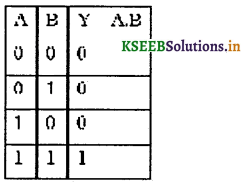Question 22.
Give the logic symbol, Boolean expression and truth table of a NOT gate?Question 23.
Give the logic symbol, Boolean expression and truth table of a NAND gate?Question 24.
Give the logic symbol, Boolean expression and truth table of a NOR gate.Question 25.
Write the Boolean equation for a NOR gate and a NAND gate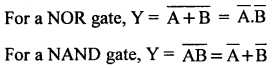Question 26.
Name the two types of integrated circuits.
The two types of ICs are

1. Linear or analogue ICs
2. Non linear or digital ICs.Question 27.
How are electron-hole pairs created in a semiconductor?
When an electron in the valence band is excited by light, heat or electric field, it gets separated from the neighbouring electron previously held by a covalent bond. The vacant site left behind acquires a positive charge and is called a hole, and with the excited electron which shifts to the conduction band forms an electron-hole pair.

Question 28.
When does electron-hole recombination take place?
When an electron in the conduction band jumps to the valence band, energy is released in the form of light or heat depending upon the energy and wavelength. Electrons occupy the vacant site and thus recombination takes place after losing the energy.

Question 29.
Give a schematic two-dimensional representation of an intrinsic Si or Ge crystal.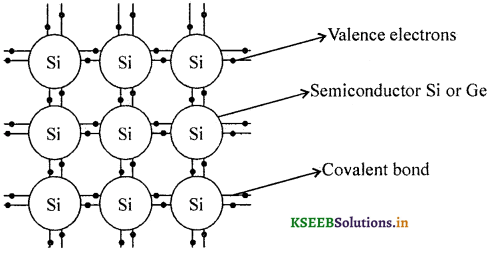Question 30.
Draw energy band diagram of an intrinsic semiconductor at room temperature.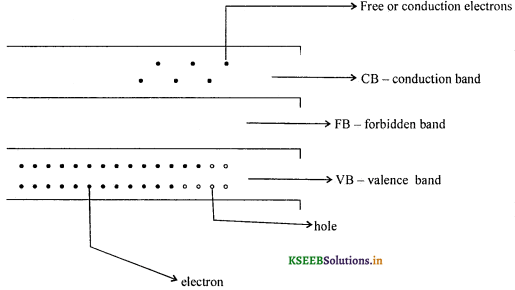We note that number of holes in the VB = Number of electrons in the CB for an intrinsic semiconductor.Question 31.
Draw I-V characteristics of a Zener diode with neat labelling.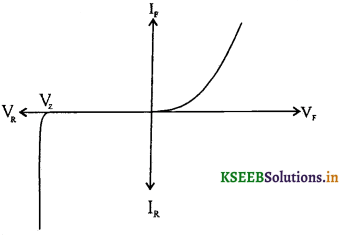Reverse curve at Vz is a vertical line parallel to the Y-axis showing that Vz remains the same for increasing reverse current in it.

Question 32.
Draw a neat labelled diagram of a Zener diode used as a DC voltage regulator.Across AB, we get a regulated voltage supply.

Question 33.
Write the symbols for a NPN and PNP transistors.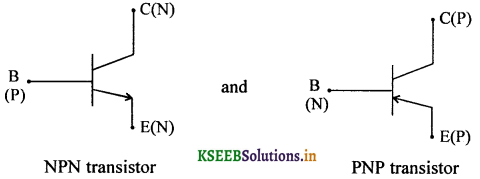Question 34.
Draw the output characteristic curve for a CE transistor circuit.Question 35.
Give the expression for voltage gain in a CE amplifier along with the meaning of the symbols used.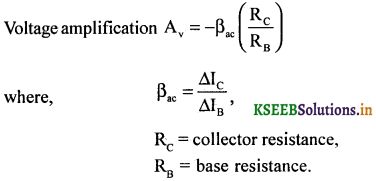Question 36.
What is a tuned circuit?
A tuned circuit is combination of capacitors and inductors. Electrostatic energy stored in the capacitor converts into electromagnetic energy in the inductor. As a result of this, the transistor is driven to saturation to cut-off and vice-versa.

Question 37.
If the following waveform is applied to an OR gate, then write the output.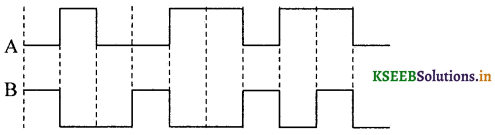Output has the digital code 110 1111110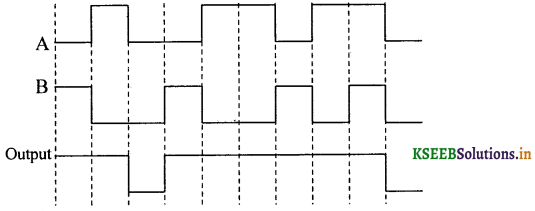### 2nd PUC Physics Semiconductor Electronics Three Marks Questions and Answers

Question 1.
What is an energy band? How are (i) Conduction band (ii) Valence band formed? (March 2014)
A collection of energy levels of electrons of a subshell is known as energy band. In the case of isolated atoms, the energy levels will be discrete. When the atoms are very close to each other as in solids, nucleus of each atom interacts with the electrons of the neighboring atoms. This splits each energy level into a number of closely packed energy levels which appear as bands.

The highest energy band which is completely filled at zero kelvin is called the valence band. The next higher band which may be partially filled or completely empty is called the conduction band.

Question 2.
What are semiconductors? Mention the two types of semiconductors.
A class of materials whose electrical conductivity lies between those of conductors and non conductors are called semiconductors.

There are two types of semiconductors namely intrinsic semiconductors and extrinsic semi conductors.

Question 3.
What is meant by doping? Mention the two types of doping agents?
The process of adding impurity to a semiconductor is called doping. The two types of doping agents are pentavalent atoms called donors like P, As, Sb, Bi and trivalent atoms called acceptors like B, Ga, In, and Al.

Question 4.
What is rectification? Mention any two types of rectifiers.
The process of converting AC into pulsating DC is called rectification. The Electronic circuits. which bring about the rectification are called rectifiers.
Two types of rectifiers are

1. Half wave rectifier
2. Full wave rectifier.Question 5.
Explain the construction and working of a light emitting diode (LED).
A light emitting diode is a p-n junction diode which emits visible light when it is forward biased.-

Working: When a LED is forward biased, recombination of electrons and holes takes place. Every recombination results in the emission of photons of energy equal to the energy gap. LED consumes very less amount of power and has a longer life. LEDs are used in 7 segment display and in traffic signal lights.

Question 6.
Explain briefly the doping level and size of the three regions of a transistor.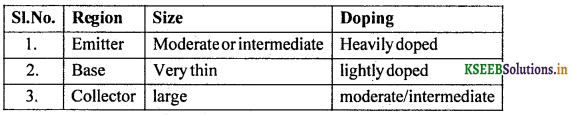Question 7.
Draw reverse bias mode connection for a photodiode circuit. How does photoelectric current depends on the intensity of incident radiation?
Photo electric current is directly proportional to intensity of radiation. Photo conduction is due
to minority charge carriers.Question 8.
Give three advantages of LEDs over conventional incandescent low power lamps.

1. Fast on-off switching capabilites.
2. From the observed wavelength in the visible range the wavelengths drifts only from – 100A to 500A. Hence the light is nearly monochromatic.
3. LEDs consumes low power, operates under a very low voltage from 1,6V to 2.2V and have a long life.

Question 9.
Write any three basic processes involed in the generation of e.m.f by a solar cell.
The three basic processes involved are,

1. Generation of electron – hole pairs due to incident light of energy greater than the forbidden gap energy.
2. Separation of electrons and holes sweeping across the junction on either side due to electric field at the depletion region.
3. Collection of electrons and holes by the two sides of semiconductors (electrons towards N – side and holes towards P – side)

Question 10.
Mention a few important criteria for the selection of a material for the fabrication of solar cells.

• Incident energy of photon should be from (1 .0eV to 1.8 eV) depending on the materials selected.
• The material should readily emit photoelectrons which depends on high optical absorption (104cm-1)
• Electrical conductivity of holes and electrons.
• Availability of raw materials and their cost.

Question 11.
Identify the terms SSI, MSI, LSI and VLSI with respect to digital ICs.

1. SSL refers to small scale integration consisting of logic gates ≤ 10
2. MSI refers to medium scale integration with logic gates ≤ 100
3. LSI refers to Large Scale Integration with logic gates ≤ 1000
4. VLSI refers to Very Large Scale Integration with logic gates > 1000.

Question 12.
Explain the working of a zener diode as a voltage regulator. (March 2014)
Circuit diagram:The circuit connections are made as shown in the figure. The circuit contains a zener diode, an unregulated voltage source (Vin > Vz), a series resistance Rs and a load resistance RL.

Any increase/decrease of voltage drop across Rs produce no change in voltage across the zener diode. For (Vin < Vz), the zener diode does not function. Thus the zener diode acts as voltage regulator.

Question 13.
Explain the working of a forward biased p-n junction diode.
Forward Bias: When a battery is connected across the junction such that p side is connected to the positive terminal and n side to the negative terminal of the source, the p-n junction is said to be forward biased. The applied voltage opposes the junction p.d. and for values of the applied voltage greater than the junction p.d. the charge carries easily across the junction and constitutes a current in an external circuit. This current rises linearly with the applied voltage beyond the knee voltage. Thus a forward biased p-n junction offers a low resistance.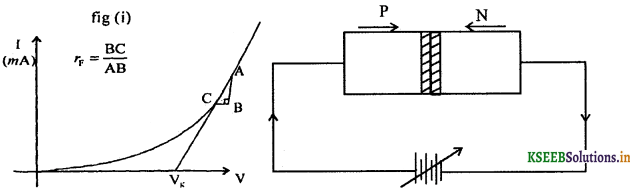Question 14.
Explain the working of a reverse biased p-n junction diode.
Reverse Bias: When battery is connected across the junction such that p side is connected to the negative terminal and n side to the positive terminal, the p-n junction is said to be reverse biased. In this case the applied voltage adds to the effect of junction p.d. and hence majority carriers cannot cross the junction. The current through the circuit is practically zero. Thus reverse bias offers a very high resistance but small current of the order of a few mA flows through the circuit due to the minority carriers in the p and n regions. As the applied voltage is gradually increased a very large current results at a particular voltage. This voltage is called the break down or cut-in voltage.### 2nd PUC Physics Semiconductor Electronics Five Marks Questions and Answers

Question 1.
What is half wave rectification? Explain the working of a half wave rectifier. Indicate the waveforms of input and output voltage.
The circuit of a half wave rectifier using a semiconductor diode is shown in figure below.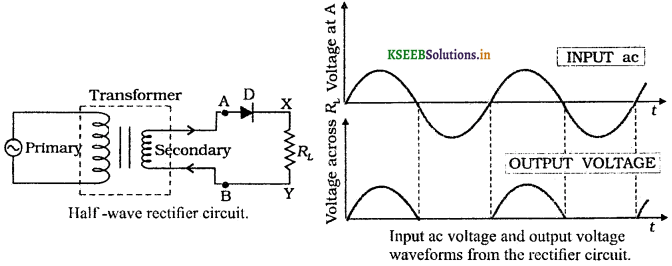The diode in series with a load resistor is connected to the secondary of a transformer. The AC voltage to be rectified is applied to the primary of the transformer.

This produces induced voltage across the secondary of the transformer. During the positive half cycles of transformer output i.e., when A is positive, diode D is forward biased and hence it conducts. During negative half cycles, the diode D is reverse biased and hence does not conduct. Current flows through the diode circuit only during positive half cycles. The current flowing through load resistance RL produces the rectified voltage.

Question 2.
What is full wave rectification? Explain the working of a full wave rectifier. Indicate the wave forms of input and output voltage. (July 2014)
Full wave rectification: The process in which both the halves of a.c. are rectified to obtain a pulsating direct current is known as full wave rectification.

A transformer with a centre tap is used for a full wave rectification. Input contains ac. A minimum of two diodes are used at the secondary of the transformer. A load resistor is connected between the junction of the N type semiconductor and the centre tap.

During the first half cycle, diode D1 will be forward biased and D2 will be reverse biased so , that the current flows from B to centre tap.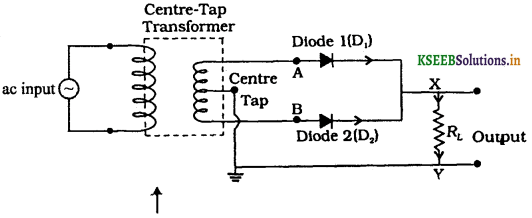During the second half cycle, diode, D2 will be forward biased while D1 will be reverse biased. The current once again takes the same direction from B to centre tap. The I/P and the O/P
waveforms are shown in fig. The average DC is $$2\left(\frac{V_{p}}{\pi}\right)$$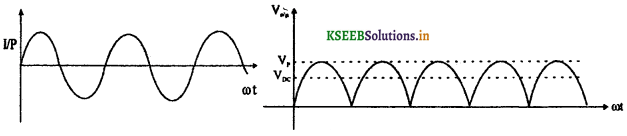Question 3.
With the help of a diagram, describe the action of a CE amplifier. (March 2015)
Ans:
1. Collector end is reverse biased and base – emitter ends forward – biased
2. A small variation in the base current produces a large variation in the collector current with a phase difference of 180° between them, without altering the input frequency. This is the basis of an amplifier circuit.
3. Power gain of the amplifier in CE mode is given by,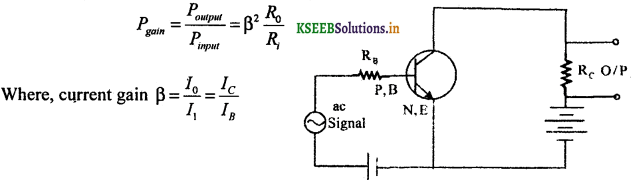4. The input and out put currents are as shown in the figure above.

Question 4.
Explain the Band theory of solids. (March 2014, 2015)
In the case of conductors, CB and VB overlap with each other. Free electrons are the charge carriers. There are a number of electrons available to conduct electricity at room temperature.

In the case of insulators there is a wide gap between CB and VB. Forbidden gap energy is greater than 5 eV. There are no electrons in the CB to conduct electricity.

In the case of semiconductors. Forbidden gap energy is less than 5 eV. There are equal number of holes and electrons in a pure semiconductor. However these charges are too low so as to conduct electricity at room temperature.Question 5.
Outline the method of drawing input and output characteristics with a neat labelled diagram of an N-P-N transistor in CE configuration.
Input characteristics:

• The voltage across the collector and emitter is maintained constant, say (VCE ≥ 5V)
• The VBB is varied and the corresponding VBE and IB (µA) are tabulated.
• A graph of IB along they – axis and VBE along the x-axis ¡s plotted. This graph is known as input characteristic curve.
• The straight line portion with a constant slope is considered to calculate static as well as dynamic input resistance.
ri = $$\left(\frac{\Delta \mathrm{V}_{\mathrm{BE}}}{\Delta \mathrm{I}_{\mathrm{B}}}\right)_{\mathrm{V}_{\mathrm{CE}}}$$ → Dynamic resistance and ri = $$\left(\frac{V_{B E}}{I_{B}}\right)_{V_{C E}}$$ → static resistance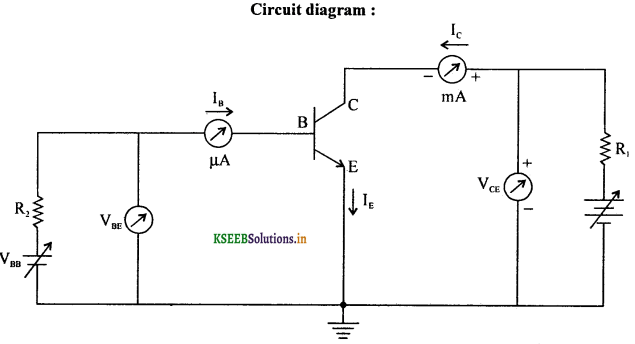Output characteristics:

• The voltage VBB is adjusted for a suitable base current (say 10 μA to 60 μA).
• ‘The voltage across VCE is varied by varying Vcc and the corresponding collector current Ic is noted.
• The results are tabulated.
• A graph of Ic (mA) along the y – axis and VCE (volts) along the x – axis is pÍotted.
• The curve obtained ¡s called output characteristics.
• The output resistance ‘r0’ is calculated by using the formula r0 = $$\left(\frac{\Delta \mathrm{V}_{\mathrm{CE}}}{\Delta \mathrm{I}_{\mathrm{C}}}\right)_{\mathrm{I}_{\mathrm{B}}}$$
Note: The ratio $$\left(\frac{\Delta \mathrm{I}_{\mathrm{C}}}{\Delta \mathrm{I}_{\mathrm{B}}}\right)_{\mathrm{V}_{\mathrm{CE}}}$$ is known as current amplification factor (β)

Question 6.
Explain briefly, the action of a transistor as a switch.• The transistor is made to function in the cut-off region and in the saturation region.
• In the above circuit, VBB = Vi = IB RB + VBE and VCE = Vcc – ICRC = V0
• The transistor will be in the cut-off region as long as the input voltage is less than the VBE = 0.65 V for silicon and 0.25 V for germanium. The transistor acts as an open switch
VB = VCC
• For a transistor operated at saturated region, VCE ≈ VCE ≈ 0. The transistor acts as a closed switch.Question 7.
Show that voltage gain in a transistor amplifier in CE mode is negative and hence obtain the expression for the voltage gain.
Circuit diagram:w.k.t DC biasing condition to be satisfied is
VBB = VBE + IB RB
When a signal voltage Vi is supplied,
Vi = ΔIBRB + ΔIBri
i.e„ Vi = ΔIB(RB + ri)
Vi = rΔIBRB where, r = RB + ri
In the amplifier circuit, for a change in base current, there will be a large variation in the collector current, i.e., ac current gainBy applying Kirchhoffs law to the output loop,
VCC = VCB + IC RL
Since change in base current causes change in collector current, ΔVCC = ΔVCE + RL ΔIC.
But ΔCC is fixed. Hence ΔVCC = 0.
ΔVCE = -RL ΔIC = V0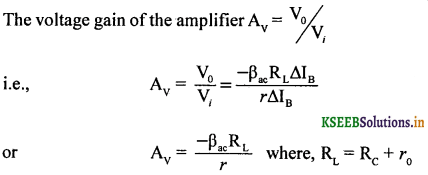Question 8.
Explain briefly the action of a transistor as a tuned collector oscillator.
Circuit diagram: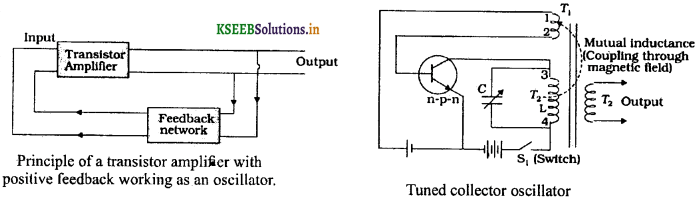Working: When the circuit is switched on, the surge current flows in the collector current. Though the current does not reach the maximum value instantaneously, it rises sinusoidally and due to feedback, current flows in the base-emitter circuit. The increase of collector current and base current will be in the same phase. Hence the nature of feedback is positive. When the current reaches the saturation point, magnetic field becomes constant. The feedback stops and hence emitter current begins to fall. This causes the magnetic field in the collector current to decay until emitter current is nearly zero. In the absence of collector current and emitter current, the transistor will be driven to cut-off. The frequency of change from saturation to cut-off is given by the formula v = $$\frac{1}{2 \pi \sqrt{L C}} \mathrm{Hz}$$Question 9.
Write a note on integrated circuits.
Integrated Circuits (ICs) are electronic devices that contain many transistors, resistors, capacitors, connecting wires on a small single silicon crystal also called as chip. These chips are miniatures measuring an area of 10-6 m2.
Integrated circuit was invented by Jack Kilky at Texas in the year 1958.
Integrated circuits are used in all the memory circuits, microprocessors, etc.

ICs are classified into linear and non-linear ICs. Operational amplifiers are linear ICs in which the output voltage varies linearly with the input.
Non – linear ICs are used in digital circuits such as logic gates. These are further classified into small scale, medium scale, large scale and very large scale integration circuits. Integrated circuits comprise of logic gates ≤ 10 to > 1000.

### 2nd PUC Physics Semiconductor Electronics List of Formula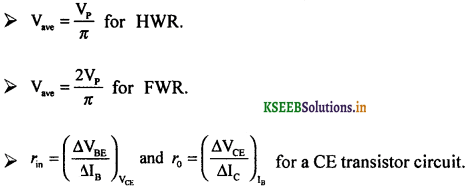### 2nd PUC Physics Semiconductor Electronics Optional Numericals and Solutions

Question 1.
If the peak value voltage in the secondary output ac voltage is 12V, then what will be the average DC in the case of HWR and FWR?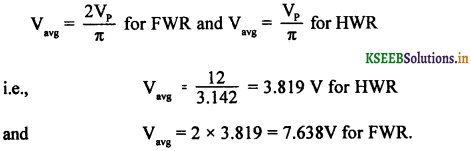Question 2.
For a CE transistor amplifier, the audio signal across the collector resistance of 2kΩ is 2V. Suppose the current amplification factor of the transistor is 100, find the input signal voltage and base current, if the base resistance is 1 kΩ.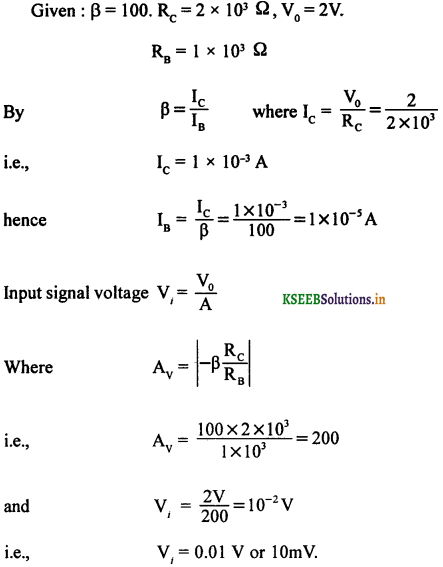Question 3.
Two amplifiers are connected one after the other in series. The first amplifier has a voltage gain of 10 and the second has a voltage gain of 20. If the input signal is 0.01V. Calculate the output ac signal.
Given Av = 10, ; A1v = 20, Vi = 0.0 1V, V0 = ?
Net voltage gain = Av A1v
= 10 × 20 = 200
By definition A = $$\frac{V_{0}}{V_{i}}$$
∴ V0 = AVi
= 200 × oov = 2.00V.

Question 4.
A p-n photo diode is fabricated from a semiconductor with band gap of 2.8eV. Can it detect a wavelength of 6000nm?
Given : λ = 6000 nm
i.e., λ = 6 × 106m
but energy E = $$\frac{h c}{\lambda}$$
$$=\frac{6.626 \times 10^{-34} \times 3 \times 10^{8}}{6 \times 10^{-6}}=3.313 \mathrm{eV}$$
Since incident energy is greater than the forbidden gap energy, photo diode can detect the radiation of wavelength 6 μm.

Question 5.
The number of silicon atoms per m3 is 5 × 1028. This is doped simultaneously with 5 × 1022 atoms per m3 of Arsenic and 5 × 1020 per m3 atoms of Indium. Calculate the number of electrons and holes given that ni = 1.5 × 1016 m-3. Is the material n-type or p-type?
Arsenic is pentavalent and Indium is trivalent.
∴ ne = 5 × 1022 – 0.05 × 1022 = 4.95 × 1022
but n2i = nenh
∴ nh = $$\frac{2.25 \times 10^{32}}{4.95 \times 10^{22}}$$ = 0.454 × 1010
nh = 4.54 × 109 per m3
since ne > nh, the material is n type.Question 6.
Calculate the voltage gain of the following common-emitter amplifier. If the input signal voltage is 20 mV then calculate the output voltage.
Circuit diagram: